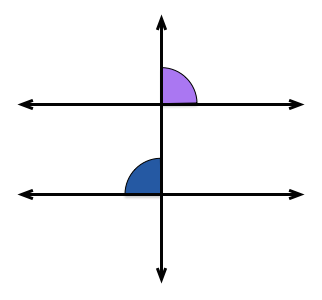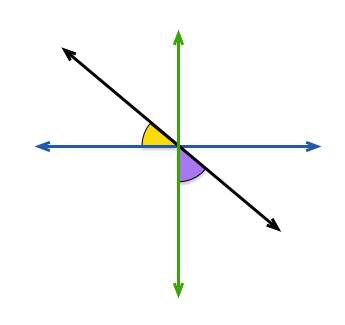Geometry

# Perpendicular LinesIf the vertical line is perpendicular to both of the horizontal lines, which of the following statements about the blue and purple angles is false?If the blue and green lines are perpendicular, which of the following statements is true about the yellow and purple angles?Given that $$\angle a = 53^\circ$$ and $$\angle b = 37^\circ$$, which of the following statements are true:

I) $$l$$ and $$m$$ are perpendicular.

II) $$l$$ and $$n$$ are perpendicular.

III) None of the lines in this diagram are perpendicular.If $$l \perp m$$ and $$n \perp p$$ and $$\angle a = 31^\circ$$, what is the measure of $$\angle b$$ in degrees?

What is the angle measure (in degrees) between 2 perpendicular lines?

×